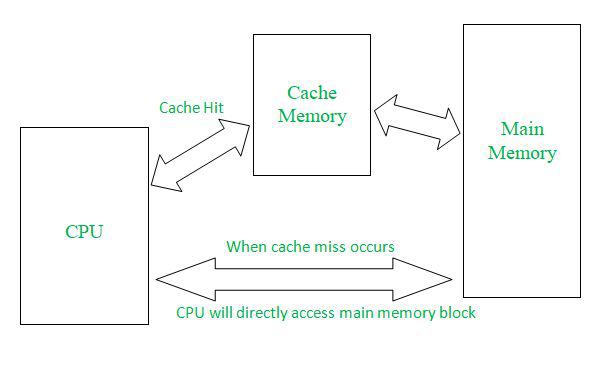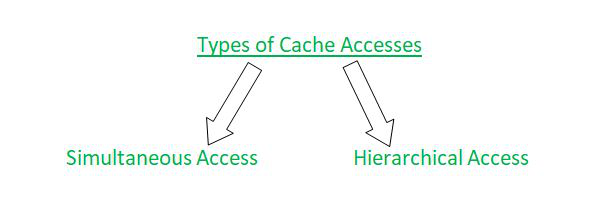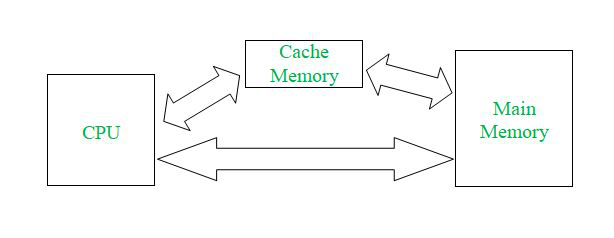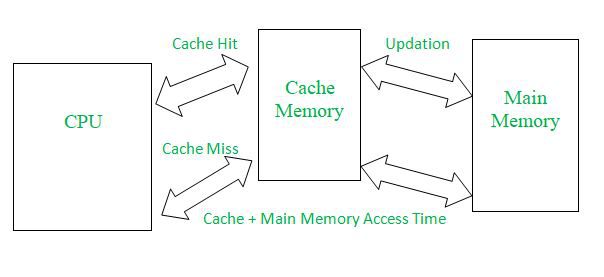# Simultaneous and Hierarchical Cache Accesses

• Difficulty Level : Basic
• Last Updated : 04 Aug, 2021

Prerequisite : Cache Organization

Introduction :
In this article we will try to understand about Simultaneous Cache access as well as Hierarchical Cache Access in detail and also understand how these access actually works whenever CPU (Central Processing Unit) requests for Main Memory Block which is being stored currently in cache memory.

Before jumping directly into the types of Cache Accesses let us first understand Cache Memory in a brief manner like what exactly Cache Memory is and why there is need to use Cache Memory.

Working of Cache Memory :Following key points (which are illustrated from the above pictorial representation) will cover briefly about Cache Memory, it’s importance and how to use it effectively –

• If CPU generates an address (which is a main memory address itself) and wishes to perform some kind of operation on the content present on that particular content, then by accessing the Main Memory directly will be a time consuming process.
• The reason for being it to be time consuming is that CPU will first check its generated address in the main memory at let us suppose if CPU find that particular address on the main memory then afterwards CPU will perform its operation over that operand (value which is present in a particular main memory address).
• This whole process will take huge amount of time which will eventually make CPU bit slow and will also cause program to damage or destroy very badly.
• That’s why we need Cache memory so as to make CPU working bit fast while accessing any sort of information which CPU will eventually receive form Cache Memory, where we keep the most demanded or needed data which CPU needs for its accessing.
• In Cache memory we will keep all the block which are needed for CPU and thus we will reduce the memory access time for accessing a particular content from the main memory since now we are accessing it via Cache Memory.
• If CPU tries to access main memory block which is not found in Cache memory, then CPU will have to access main memory itself for further operations which are to be performed on a particular main memory block.

Now that you have understood the basic concept of Cache Memory and how does this work whenever CPU wants to access any main memory address, let us jump into our main topic of consideration which is Simultaneous Cache Access and Hierarchical Cache Access.

Types of Cache Accesses :There are two types of Cache Accesses possible whenever CPU wishes to access a particular main memory address: Simultaneous Cache Access and Hierarchical Cache Access. Both of them have similar kind of block representation but their working, accessing and most importantly their average memory access time is different respectively.

1. Simultaneous Cache Access :• In simultaneous cache access, request for cache memory and main memory are generated simultaneously.
• If CPU generated address is found in Cache Memory then it is considered as Hit and then CPU could directly access the main memory address as well as it contents from cache memory itself and further if any sort of changes happens in Cache memory with respect of that particular then that changes will be done in Main Memory also after Cache Memory.
• If CPU generate address is not found in Cache Memory then it is considered as Miss and then CPU has to access that address from main memory and further more that block from main memory is moved to Cache Memory for future references as well as usage.
• Simultaneous Memory Access is used for the implementation of Write Through Cache.

Calculation of Average Memory Access Time in Simultaneous Access –
If its a hit then CPU will access content from cache memory itself and if its a miss then therefore Main Memory will come into action.

Therefore Average memory access time in case of Simultaneous Access will be shown below –

• Average Memory Access Time = Hit ratio * Cache Memory Access Time + (1 – Hit ratio) * Main Memory Access Time

If suppose locality of reference is included here, then we use following concept to determine the Average memory access time –

• Average Memory Access Time = Hit ratio * Cache Memory Access Time + (1 – Hit ratio) * Time required to access a block of main memory.
where, Time required to access a main memory block = block size * Time required to access main memory.

If suppose block transfer time is also included here, then we use following concept to determine the Average memory access time –

• Average Memory Access Time = Hit ratio * Cache Memory Access Time + (1 – Hit ratio) * (Cache Memory Acces Time + Time required to access a block of main memory)
where, Time required to access a main memory block = block size * Time required to access main memory

Here the reason for using Cache Memory Access in case of miss along with block access time is that we need to copy the block into Cache Memory (if illustrated in any particular scenario).

2. Hierarchical Cache Access :Although basic block structure remains similar to that of Hierarchical Cache Access but there are actually several differences between both Hierarchical and Simultaneous Cache memory accesses which are described below (in points)-

• In hierarchical cache access only the faster memory (which is Cache memory) is accessed first.
• Afterwards if address generated by CPU is not found in Cache memory then along with searching time in Cache memory main memory access time will also be counted.
• By default many computer designs are made using this hierarchical access only because this cache access reduces average memory access time due to locality of reference concept (in which for a particular address of main memory we just bring its previous and its next address also in form of block so that in future less memory access is required).

Calculation of of Average Memory Access Time in Hierarchical Access –

If its a hit then CPU will access content from cache memory itself and if its a miss then Along with Cache Memory Access Time Main Memory Access Time is also counted.
Therefore Average memory access time in case of Hierarchical Access will be shown below –

• Average Memory Access Time = Hit ratio * Cache Memory Access Time + (1 – Hit ratio) * (Cache Memory Access Time + Main Memory Access Time)

Example 1 –
In 2-level hierarchy, if the top level has an access time of 10ns and the bottom level has an access time of 60ns, what is the hit rate on the top level required to give an average access time of 15ns?

Explanation –
According to example, we have been given Cache Access time (top level) = 10ns and Main memory access access time (bottom level)= 60ns and Average memory access time = 15ns.

Therefore we will apply the above illustrated concept of hierarchical access(because in question it was written 2-level hierarchy) for calculating the memory access time which-
Average memory access time = Hit ratio * Cache memory access time + (1 – Hit ratio) * (Cache Memory access time + Main memory access time)
15ns = 10 * Hit ratio + (1 – Hit ratio) * (10ns + 60ns)
15ns-70ns = -60 * Hit ratio
Hit ratio = 55 /60 = 11/12 = 0.9 (Ans.)
If suppose locality of reference is included here, then we use following concept to determine the Average memory access time.

• Average Memory Access Time = Hit ratio * Cache Memory Access Time + (1 – Hit ratio) * (Time required to access a block of main memory + Cache memory access time)
where, Time required to access a main memory block = block size * Time required to access main memory

Example 2 –
In two level hierarchy, the top level has an access time of 10ns and bottom level has an access time of 50ns, the hit rate on the top level is 90%. If the block size of cache is 16 bytes, then what is the average memory access time required? (Consider the system uses locality of reference)

Explanation –
Given Cache memory access time (top level)= 10ns
Main memory access time (bottom level) = 60ns
Therefore we will apply the above learnt concept for calculating the average memory access time when we have to consider locality of reference concept into consideration.

• Average memory access time = Hit ratio * Cache memory access time + (1 – Hit ratio) * ((Block size * main memory access time) + cache memory access time)

Average memory access time = 10 * 0.9 + (1 – 0.9) * (16 * 5 + 10)
= 9 + (0.1) * (800 + 10)
= 9 + 81 = 90ns (Ans.)
If suppose block transfer time is also included here, then we use following concept to determine the Average memory access time.

Average Memory Access Time = Hit ratio * Cache Memory Access Time + (1 – Hit ratio) * (Cache Memory Access Time + Time required to access a block of main memory + Cache Memory access time)
where, Time required to access a main memory block = block size * Time required to access main memory

Example 3 –
In two level hierarchy, the cache has an access time of 12ns and the main memory access time of 120ns, the hit rate of cache is 90%. If the block size of cache is 16 bytes then what is the average memory access time including Miss Penalty? (Miss Penalty: Time to bring main memory block to cache memory when cache miss occurs)
Explanation –
Given Cache memory access time= 12ns
Main memory access time= 120ns
Therefore we will use the above learnt concept for calculating the average memory access time when block transfer occurs from main memory to cache memory on cache miss.

• Average memory access time = Hit ratio * Cache memory access time + (1 – Hit ratio) * (Cache memory access time + (Block size * Main memory access time)+ Cache memory access time due to block transfer)

Average memory access time = 0.9 * 12 = (0.1) * (12ns + 16 * 120 ns + 12ns)
= 10.8ns + 194.4ns = 205.2ns (Ans.)

My Personal Notes arrow_drop_up